## Dielectric constant of a plasma

A plasma is very similar to a gaseous medium, expect that the electrons are free: i.e., there is no restoring force due to nearby atomic nuclii. Hence, we can obtain an expression for the dielectric constant of a plasma from Eq. (1149) by settingto zero, andto the number density of electrons,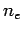. We obtain(1150)

where the characteristic frequency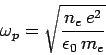(1151)

is called the plasma frequency. We can immediately see that formula (1150) is problematic. For frequencies above the plasma frequency, the dielectric constant of a plasma is less than unity. Hence, the refractive index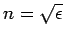is also less than unity. This would seem to imply that high frequency electromagnetic waves can propagate through a plasma with a velocity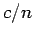which is greater than the velocity of light in a vacuum. Does this violate the principles of relativity? On the other hand, for frequencies below the plasma frequency, the dielectric constant is negative, which would seem to imply that the refractive indexis imaginary. How should we interpret this?

Consider an infinite plane-wave, of frequency,, greater than the plasma frequency, propagating through a plasma. Suppose that the wave electric field takes the form(1152)

where it is understood that the physical electric field is the real part of the above expression. A peak or trough of the above wave travels at the so-called phase velocity, which is given by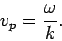(1153)

Now, we have also seen that the phase velocity of electromagnetic waves in a dielectric medium is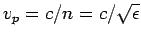, so(1154)

It follows from Eq. (1150) that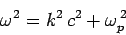(1155)

in a plasma. The above type of expression, which effectively determines the wave frequency,, as a function of the wave-number,, for the medium in question, is called a dispersion relation (since, amongst other things, it determines how fast wave-pulses disperse in the medium). According to the above dispersion relation, the phase velocity of high frequency waves propagating through a plasma is given by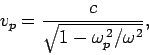(1156)

which is indeed greater than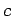. However, the theory of relativity does not forbid this. What the theory of relativity says is that information cannot travel at a velocity greater than. And the peaks and troughs of an infinite plane-wave, such as (1152), do not carry any information.

We now need to consider how we could transmit information through a plasma (or any other dielectric medium) by means of electromagnetic waves. The easiest way would be to send a series of short discrete wave-pulses through the plasma, so that we could encode information in a sort of Morse code. We can build up a wave-pulse from a suitable superposition of infinite plane-waves of different frequencies and wave-lengths: e.g.,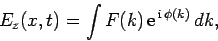(1157)

where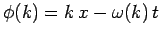, and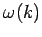is determined from the dispersion relation (1155). Now, it turns out that a relatively short wave-pulse can only be built up from a superposition of plane-waves with a relatively wide range of differentvalues. Hence, for a short wave-pulse, the integrand in the above formula consists of the product of a fairly slowly varying function,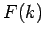, and a rapidly oscillating function,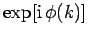. The latter function is rapidly oscillating because the phase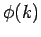varies very rapidly with, relative to. We expect the net result of integrating the product of a slowly varying function and rapidly oscillating function to be small, since the oscillations will generally average to zero. It follows that the integral (1157) is dominated by those regions of-space for whichvaries least rapidly with. Hence, the peak of the wave-pulse most likely corresponds to a maximum or minimum of: i.e.,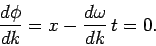(1158)

Thus, we infer that the velocity of the wave-pulse (which corresponds to the velocity of the peak) is given by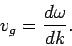(1159)

This velocity is called the group velocity, and is different to the phase velocity in dispersive media, for whichis not directly proportional to. (Of course, in a vacuum,, and both the phase and group velocities are equal to.) The upshot of the above discussion is that information (i.e., an individual wave-pulse) travels through a dispersive media at the group velocity, rather than the phase velocity. Hence, relativity demands that the group velocity, rather than the phase velocity, must always be less than.

What is the group velocity for high frequency waves propagating through a plasma? Well, differentiation of the dispersion relation (1155) yields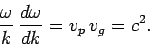(1160)

Hence, it follows from Eq. (1156) that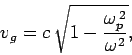(1161)

which is less than. We thus conclude that the dispersion relation (1155) is indeed consistent with relativity.

Let us now consider the propagation of low frequency electromagnetic waves through a plasma. We can see, from Eqs. (1156) and (1161), that when the wave frequency,, falls below the plasma frequency,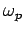, both the phase and group velocities become imaginary. This indicates that the wave attenuates as it propagates. Consider, for instance, a plane-wave of frequency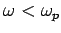. According to the dispersion relation (1155), the associated wave-number is given by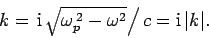(1162)

Hence, the wave electric field takes the form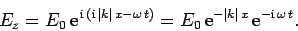(1163)

Indeed, it can be seen that forelectromagnetic waves in a plasma take the form of decaying standing waves, rather than traveling waves. We conclude that an electromagnetic wave, of frequency less than the plasma frequency, which is incident on a plasma will not propagate through the plasma. Instead, it will be totally reflected.

We can be sure that the incident wave is reflected by the plasma, rather than absorbed, by considering the energy flux of the wave in the plasma. It is easily demonstrated that the energy flux of an electromagnetic wave can be written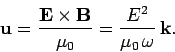(1164)

For a wave with a real frequency and a complex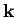-vector, the above formula generalizes to(1165)

However, according to Eq. (1162), the-vector for a low frequency electromagnetic wave in a plasma is purely imaginary. It follows that the associated energy flux is zero. Hence, any low frequency wave which is incident on the plasma must be totally reflected, since if there were any absorption of the wave energy then there would be a net energy flux into the plasma.

The outermost layer of the Earth's atmosphere consists of a partially ionized zone known as the ionosphere. The plasma frequency in the ionosphere is about 1MHz, which lies at the upper end of the medium-wave band of radio frequencies. It follows that low frequency radio signals (i.e., all signals in the long-wave band, and most in the medium-wave band) are reflected off the ionosphere. For this reason, such signals can be detected over the horizon. Indeed, long-wave radio signals reflect multiple times off the ionosphere, with very little loss (they also reflect multiple times off the Earth, which is enough of a conductor to act as a mirror for radio waves), and can consequently be detected all over the world. On the other hand, high frequency radio signals (i.e., all signals in the FM band) pass straight through the ionosphere. For this reason, such signals cannot be detected over the horizon, which accounts for the relatively local coverage of FM radio stations. Note, from Eq. (1151), that the plasma frequency is proportional to the square root of the number density of free electrons. Now, the level of ionization in the ionosphere is maintained by ultra-violet light from the Sun (which effectively knocks electrons out of neutral atoms). Of course, there is no such light at night, and the number density of free electrons in the ionosphere consequently drops as electrons and ions gradually recombine. It follows that the plasma frequency in the ionosphere also drops at night, giving rise to a marked deterioration in the reception of distant medium-wave radio stations.